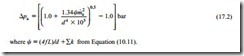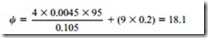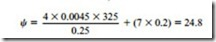# Coarse material:Procedure and Operating point.

##### Procedure

The location of the equivalent operating point on the conveying characteristics for the test pipeline needs to be established first, taking account of the pressure and air flow rate requirements. Scaling is conveniently carried out in two stages. In the first stage scaling is with respect to conveying distance, and this includes both pipeline orientation and bends. In the second stage the scaling is with respect to pipeline bore. Air only pressure drop values need to be established and so this procedure is also included.

###### Operating point

The operating point on the conveying characteristics for the test pipeline on Figure 17.2 must first be identified. Since the pressure drop line has been chosen as 0.85 bar and the conveying line inlet air velocity has been determined as 17 m/s, the appropriate air mass flow rate can be calculated. This can be determined from Equation (13.1), reproduced here as Equation (17.1) for reference and use:

where . is the air mass flow rate (kg/s); p , the conveying line inlet air pressure (185 kN/m2 abs); d, the pipeline bore (0.105 m); C1, the conveying line inlet air velocity (17 m/s) and T1, the conveying line inlet air temperature (288 K (15°C)).

Substituting these values in Equation (17.1) gives ma = 0.330 kg/s

This operating point is located on Figure 17.2 as point (a) and it will be seen that it is approximately 20 per cent in-board from the conveying limit.

###### Air only pressure drop values

The air only pressure drop for a pipeline, pa , can be determined using Equation (10.14), reproduced here as Equation (17.2).Taking the pipeline friction factor, f, to be 0.0045, the length of the test pipeline, L, as 95 m, the pipeline bore, d, as 0.105 m, and the bend loss coefficient as 0.2 (Figure 10.6) for each of nine bends, gives:Substituting this value, the air flow rate of 0.330 kg/s and the pipeline bore of 0.105 m into Equation (17.2) gives:

pa = 0.103 bar

From this it will be seen that the air only pressure drop is quite significant for dilute phase flow. This value of pressure drop is automatically included in the conveying characteristics in Figure 17.2. A constant pressure drop line of 0.103 bar, if included on the plot, would strike the horizontal axis at an air flow rate of 0.330 kg/s. It also means that at the operating point only 0.850-0.103 = 0.747 bar is used for conveying material. This value will decrease with increase in pipeline length.

###### Plant pipeline of 105 mm bore

The actual length of the plant pipeline is 325 m and it is this length that needs to be used to evaluate the air only pressure drop for the plant pipeline having the same bore as the test pipeline. Taking the pipeline friction factor, f, to be 0.0045, the length of the plant pipeline, L, as 325 m, the pipeline bore, d, as 0.105 m and the bend loss coeffi- cient as 0.2 (Figure 10.6) for each of seven bends, gives:

Substituting this value, the air flow rate of 0.330 kg/s and the pipeline bore of 0.105 m into Equation (17.2) gives:

pa = 0.298 bar

This represents an increase in air only pressure drop of 0.298 – 0.103 = 0.195 bar. This means that instead of having 0.747 bar for conveying material, it is now reduced to 0.747 – 0.195 = 0.552 bar. This represents a 26 per cent reduction in available pressure drop and so this will have a very significant effect on the material flow rate that can be achieved. This is in addition to the reduction as a consequence of scaling to a longer pipeline.

To achieve the 15 tonne/h in the plant pipeline, however, a much larger bore pipeline will be required and this will improve the situation considerably. When the conveying characteristics are scaled in total these features can be seen, as with Figures 14.1, 14.4

and 14.5. When only a single point is used the intermediate stage of the data scaled to the plant pipeline, of the test pipeline bore, is not available. This means that a value for the plant pipeline bore needs to be selected at this point. If the value chosen does not meet the required duty the calculation will have to return to this point with an updated value. For 15 tonne/h a bore of 250 mm will be selected.

###### Taking the pipeline friction factor, f, to be 0.0045, the length of the plant pipeline, L, as 325 m, the pipeline bore, d, as 0.250 m and the bend loss coefficient as 0.2 (Figure 10.6) for each of seven bends, gives:For the larger bore of pipeline a new air flow rate will be required. This can either be determined by using Equation (17.1), as for the test pipeline, or scaling the 0.330 kg/s for the test pipeline in proportion to the larger pipe section area. Either way the new air flow rate will come to 1.87 kg/s.

Substituting the new value for , the new air flow rate of 1.87 kg/s and the pipeline bore of 0.250 m into Equation (17.2) gives:

pa = 0.139 bar

The original operating point on the material conveying characteristics on Figure 17.2 was set at a pressure drop of 0.85 bar (point a). For the plant pipeline the air only pres- sure drop is 0.139 bar whereas for the test pipeline it is 0.103 bar, which represents an increase of 0.036 bar. The operating point on Figure 17.2 therefore needs to be reduced by this amount for scaling purposes, to take account of the difference in air only pressure drop values. The new operating point (b) is therefore at a pressure drop of 0.814 bar.

###### Equivalent lengths

The equivalent length of a pipeline for the conveying of material takes the length of horizontal pipeline as the reference value. To this is added an equivalent length of straight horizontal pipeline, both for the vertically up sections of pipeline and for the bends in the pipeline. These two elements were considered in Chapter 14 on ‘Pipeline scaling parameters’. This procedure was considered at this point in the previous case study and an expression for the equivalent length, Le, of a pipeline was given with Equation (16.3). This is reproduced here as Equation (17.3) for use in this case study:

where h is the total length of horizontal sections of pipeline; v, the total length of vertically up sections of pipeline; N, the total number of bends in pipeline and b, the equiva- lent length of each bend.

A sketch of the test pipeline is given in Figure 17.1 and from this it will be seen that the equivalent length of the test pipeline, Le1, is:

There is no significant vertical lift and there are nine bends in the test pipeline. With a conveying line inlet air velocity of 17 m/s the equivalent length of the bends, from Figure 17.3, is about 20 m each. It will be seen from this that the bends can have a dominating effect in dilute phase conveying systems.

###### Plant pipeline

The equivalent length of the plant pipeline, Le2, with 300 m of horizontal pipeline, 25 m of vertical pipeline and seven 90° bends is:

###### Scaling

The data for the test pipeline can now be scaled to that for the plant pipeline. The first stage is in terms of equivalent length and the second in terms of pipeline bore.

###### Scaling for length

The scaling model for pipeline length is given in Equation (14.4) and is reproduced here in Equation (17.4):

The two equivalent lengths were determined immediately above, and the material flow rate for the test pipeline of 5.65 tonne/h was obtained from the revised operating point on Figure 17.2; 3.17 tonne/h is the material flow rate that would be expected, for the same conveying line pressure drop and air flow rate, if the pipeline had the same bore as the test pipeline.

###### Scaling for bore

A scaling model for pipeline bore is given in Equation (14.8). This is reproduced here as Equation (17.5) for application in this case:

It is the 3.17 tonne/h that needs to be scaled here and substituting data into this equa- tion gives:

This is greater than the 15 tonne/h required, but significantly less than 15 tonne/h would be achieved with a smaller 200 mm bore pipeline. A pressure greater than 1.0 bar would be needed if it was required to use a 200 mm bore pipeline, but then it would not be pos- sible to use a positive displacement blower.

With a conveying line inlet air pressure of 0.85 bar gauge the case for stepping the pipeline to a larger bore is marginal. Little improvement in conveying performance would be achieved, but it would certainly help if there was a need to reduce erosive wear of particle degradation.

###### Air requirements

An air supply pressure of 0.85 bar gauge was selected at the outset and so the free air flow rate and an approximate value for the power supply are now required.

###### Air flow rate

The air flow rate will be evaluated for the 250 mm bore pipeline, assuming that the air supply pressure will be about 0.85 bar gauge. The equations for evaluating air flow rate were developed in Chapter 9. The design here is based on a conveying line inlet air velocity of 17 m/s and Equation (9.10), reproduced here as Equation (17.6) is appropriate:

This is the volumetric flow rate of the air at free air conditions, which are the reference conditions required for the specification of a compressor.

###### Power required

An approximate value for the compressor drive power required was presented in Equation (6.5) and this is reproduced here as Equation (17.7):

Specific cost

Pneumatic conveying, and particularly dilute phase conveying, does require high energy levels. The cost of transporting material, therefore, is often taken into account when selecting a conveying system. With an estimated value for power required it is possible to evaluate conveying costs.

If the unit cost of electricity is taken as €0.10/kW · h the specific cost can be

evaluated as follows: